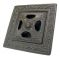##### The Japanese Bonsai specialist
Direct order Contact Help / Services Newsletter# Ashtray a57

› Bonsai tables and jita › Japanese craftsref. : 4478

55,00

Available quantity : 1Order

###### Description

Ashtray in melting. Dimensions:224*224*46 mm. Made in melting in traditional traditional foundry in the Japanese region of Nanbu. Article of quality, removable lid.

#japanese 3.8 #ashtray 3.7 #traditional 3.1 #melting 2.7 #crafts 2.6 #dimensions 2 #removable 1.9 #article 1.7 #foundry 1.7 #quality 1.7

Formule
(( ROUND((CHAR_LENGTH(b.article_nom)-CHAR_LENGTH(REPLACE(b.article_nom, 'ashtray', '')))/LENGTH('ashtray')) + ROUND((CHAR_LENGTH(b.article_description)-CHAR_LENGTH(REPLACE(b.article_description, 'ashtray', '')))/LENGTH('ashtray')) ) * 3.7) + (( ROUND((CHAR_LENGTH(b.article_nom)-CHAR_LENGTH(REPLACE(b.article_nom, 'traditional', '')))/LENGTH('traditional')) + ROUND((CHAR_LENGTH(b.article_description)-CHAR_LENGTH(REPLACE(b.article_description, 'traditional', '')))/LENGTH('traditional')) ) * 3.1) + (( ROUND((CHAR_LENGTH(b.article_nom)-CHAR_LENGTH(REPLACE(b.article_nom, 'melting', '')))/LENGTH('melting')) + ROUND((CHAR_LENGTH(b.article_description)-CHAR_LENGTH(REPLACE(b.article_description, 'melting', '')))/LENGTH('melting')) ) * 2.7) + (( ROUND((CHAR_LENGTH(b.article_nom)-CHAR_LENGTH(REPLACE(b.article_nom, 'dimensions', '')))/LENGTH('dimensions')) + ROUND((CHAR_LENGTH(b.article_description)-CHAR_LENGTH(REPLACE(b.article_description, 'dimensions', '')))/LENGTH('dimensions')) ) * 2) + (( ROUND((CHAR_LENGTH(b.article_nom)-CHAR_LENGTH(REPLACE(b.article_nom, 'removable', '')))/LENGTH('removable')) + ROUND((CHAR_LENGTH(b.article_description)-CHAR_LENGTH(REPLACE(b.article_description, 'removable', '')))/LENGTH('removable')) ) * 1.9) + (( ROUND((CHAR_LENGTH(b.article_nom)-CHAR_LENGTH(REPLACE(b.article_nom, 'japanese', '')))/LENGTH('japanese')) + ROUND((CHAR_LENGTH(b.article_description)-CHAR_LENGTH(REPLACE(b.article_description, 'japanese', '')))/LENGTH('japanese')) ) * 1.8) + (( ROUND((CHAR_LENGTH(b.article_nom)-CHAR_LENGTH(REPLACE(b.article_nom, 'article', '')))/LENGTH('article')) + ROUND((CHAR_LENGTH(b.article_description)-CHAR_LENGTH(REPLACE(b.article_description, 'article', '')))/LENGTH('article')) ) * 1.7) + (( ROUND((CHAR_LENGTH(b.article_nom)-CHAR_LENGTH(REPLACE(b.article_nom, 'foundry', '')))/LENGTH('foundry')) + ROUND((CHAR_LENGTH(b.article_description)-CHAR_LENGTH(REPLACE(b.article_description, 'foundry', '')))/LENGTH('foundry')) ) * 1.7) + (( ROUND((CHAR_LENGTH(b.article_nom)-CHAR_LENGTH(REPLACE(b.article_nom, 'quality', '')))/LENGTH('quality')) + ROUND((CHAR_LENGTH(b.article_description)-CHAR_LENGTH(REPLACE(b.article_description, 'quality', '')))/LENGTH('quality')) ) * 1.7) + (( ROUND((CHAR_LENGTH(b.article_nom)-CHAR_LENGTH(REPLACE(b.article_nom, 'region', '')))/LENGTH('region')) + ROUND((CHAR_LENGTH(b.article_description)-CHAR_LENGTH(REPLACE(b.article_description, 'region', '')))/LENGTH('region')) ) * 1.6)

## Secure payment## Delivery

Our logistic partners :